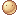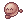# What is 2 and 2 put togeher?

+8 votes
287 views
asked Oct 2, 2019 in Other
I would like to see how many views this gets for this question.
commented Oct 3, 2019 by SnickerDoodles
PANT,PANT! I DON"T KNOW!!!!!!!!!!!!!!!!!!!!!!!!!!!!!!!!!!!!!!!!!!
commented Oct 3, 2019 by username

4 i think

commented Oct 4, 2019 by SnickerDoodles
nah, it's not 4. it's 222222222222222222222222222222222222222222222222222222222222222222222222222222222222222222222222222222222222222222222222222222222222222222222222222222222222222222222222222222222222222222222222222222222222222222222222222222222222222

## 9 Answers

+3 votes
answered Oct 10, 2019 by someone

Best answer

Well... if x = 2 and x= pi and pi= 3.14159.., then it is 2+2 = x+x= Pi+Pi= 3.14159... + 3.14159... but 22 = 4, but u never know if there is a secret 2 hiding behind the plus so, 2+2+2= 6, 222= 484, 222= 8,388,608, and that’s three equations so triangle has three pointy bits and the angle of a triangle is 60* so you need to go:

8388608 % 484= 17,331.83471074380165, and then 60%6= 10 so we go, 10x17,331.83471074380165= 173,318.34710743801653. But we round to the 3 d.p which is 173,318.347. Now we subtract 4. 173,314.347. Then I count up how many ‘4’ I can make w. 173,314.347 cos 4 is a very special number in this certain equation. There are 4 ‘4’s but if I add 7+7=14 so there is 5 ‘4’s and then 1+3=4 so 6 ‘4’s and 6 ‘4’s equal 24 and 24 x Pi is the same as 24 x 3.14159 so it equals not ‘Pi Pi Pi Pi’ but 75.39822368615504. But that number is too big for ur maths teacher so to make him/her/unidentifiedalien happy you round to 2 d.p. So you get 75.39 and carry the one=

75.4!

Me smrt.

commented Oct 10, 2019 by (123,410 points)
0.0 that's a lotta stuff for 2 and 2 put together. Wow. Good job.
commented Oct 10, 2019 by (320,940 points)
OMG!! GREAT JOB!
commented Oct 10, 2019 by someone
me smrt
commented Nov 6, 2019 by unicorn girl

Me:Her:commented Feb 3, 2020 by (21,900 points)
Ummmmm... first, that was a lot. Second, "Me smrt"

is very informal. And that is not how you spell smart.
commented Aug 14, 2020 by (52,490 points)
I am so smart

s m r t

I mean s m a r t
commented Aug 15, 2020 by (1,890 points)
im a math dyslexic so you actually look like a god to me
commented Aug 25, 2020 by (123,410 points)
Hey welshterriers, its a joke
commented Nov 27, 2020 by (21,900 points)
I know hahaha I was a hothead then. I was being stupid.
commented Nov 27, 2020 by (123,410 points)
lol
0 votes
answered Aug 13, 2020 by anonymous
22
+2 votes
answered Oct 10, 2019 by (290,180 points)
4, 22, or 222222222222222222222222222222
commented Oct 11, 2019 by SnickerDoodles
lol
+2 votes
answered Oct 10, 2019 by \$100000 dude
1. You spelt together wrong.

2. Its 5. Definately.
commented Oct 10, 2019 by (123,410 points)
I just now noticed togeher. Thx
+3 votes
answered Oct 9, 2019 by (68,710 points)
+5 votes
answered Oct 9, 2019 by (122,450 points)
The answer is fish, y’all. try it. turn the two’s on their sides
+5 votes
answered Oct 3, 2019 by (57,340 points)
Huh? 2 plus 2? UMM..........
+4 votes
answered Oct 3, 2019 by (373,980 points)
I think it's 22?
+5 votes
answered Oct 3, 2019 by (424,860 points)
You’ll probably get quite a bit